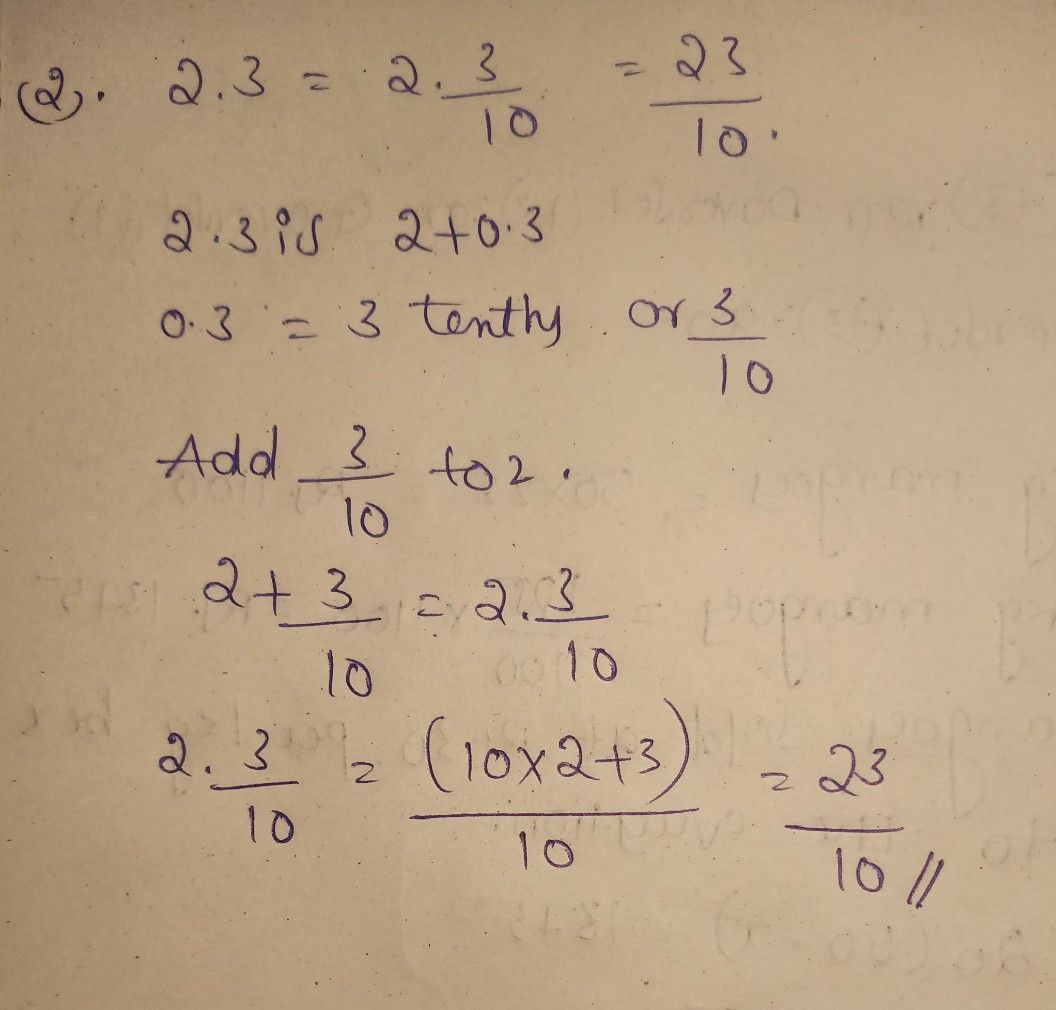Symbol
ProblemWhat I Can Do $1c\times p^{1}$ Express the Rational numbers from Froction form to Decimal $fcr0$ $an$ vice verss. $1$ What is0.75 in fraction? $2$ What $1a2.333$ in fraction? $3$ Hailey $odc$ her bike $s$ a mile to the nature park, What is the distance she rode her bilke $1cc$ as a decimal number? 2. 4. Sarah $oolc-o1$ the ham and pineapple pizza. How much of the pizza did Sarah $1a1lca$ written as a decimal number? $5$ Erik $01$ of the questions correct on the test. How is that fraction written as a decimal numnber?
7th-9th grade
Other
Search count: 106
SolutionQanda teacher - nasreensk